# Java按值传递还是引用传递？

Java总是采用按值调用。方法得到的是所有参数值的一个拷贝，特别的，方法不能修改传递给它的任何参数变量的内容。

1. 基本数据类型为参数

public class ParamTest { public static void main(String[] args) { int price = 5; doubleValue(price); System.out.print(price); } public static void doubleValue(int x) { x = 2 * x; } }

【输出结果】: 5

x被初始化为price值的一个拷贝，即5

x乘以2后等于10。但是price没有变化，依然是5

doubleValue执行完后，参数变量不再使用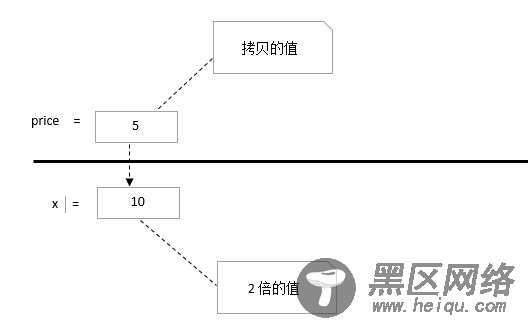2. 对象引用为参数

class Student { private float score; public Student(float score) { this.score = score; } public void setScore(float score) { this.score = score; } public float getScore() { return score; } } public class ParamTest { public static void main(String[] args) { Student stu = new Student(80); raiseScore(stu); System.out.print(stu.getScore()); } public static void raiseScore(Student s) { s.setScore(s.getScore() + 10); } }

【运行结果】：

90.0

s被赋予stu值的拷贝，这里是一个对象的引用

raiseScore方法应用于这个应用。s和stu指向同一对象，该对象的分数增加了10

raiseScore方法结束后，s不再使用，stu指向的那个对象分数增加了10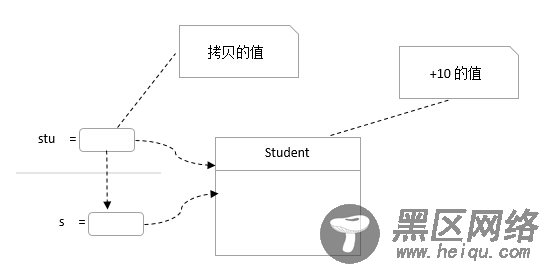3. 对对象是值调用还是引用传递？

public static void swap(Student x, Student y) { Student temp = x; x = y; y = temp; }

class Student { private float score; public Student(float score) { this.score = score; } public void setScore(float score) { this.score = score; } public float getScore() { return score; } } public class ParamTest { public static void main(String[] args) { Student a = new Student(0); Student b = new Student(100); System.out.println("交换前："); System.out.println("a的分数：" + a.getScore() + "--- b的分数：" + b.getScore()); swap(a, b); System.out.println("交换后："); System.out.println("a的分数：" + a.getScore() + "--- b的分数：" + b.getScore()); } public static void swap(Student x, Student y) { Student temp = x; x = y; y = temp; } }

【运行结果】：

a的分数：0.0--- b的分数：100.0

a的分数：0.0--- b的分数：100.0

swap方法体完成x,y的的交换，此时a,b并没有变化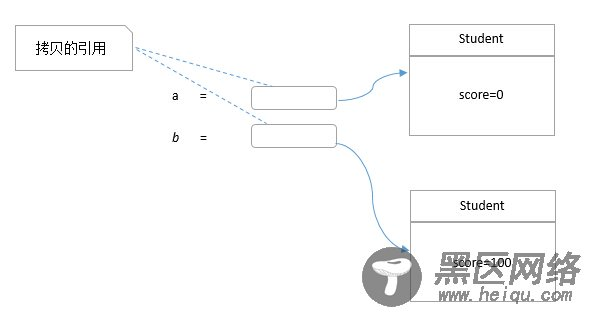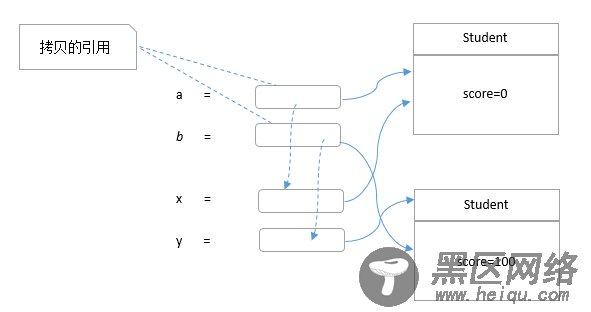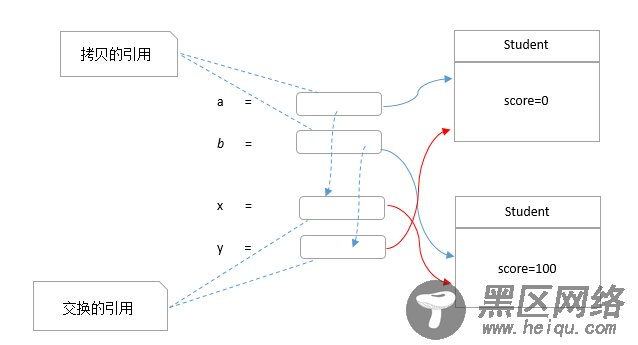swap执行完成，x,y不再使用,回到创建时状态。